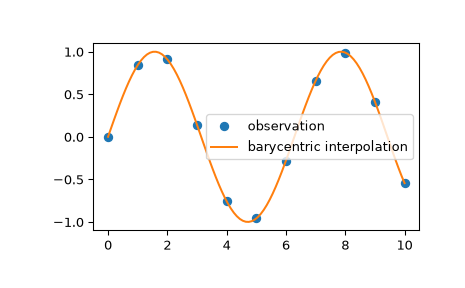# scipy.interpolate.barycentric_interpolate¶

scipy.interpolate.barycentric_interpolate(xi, yi, x, axis=0)[source]

Convenience function for polynomial interpolation.

Constructs a polynomial that passes through a given set of points, then evaluates the polynomial. For reasons of numerical stability, this function does not compute the coefficients of the polynomial.

This function uses a “barycentric interpolation” method that treats the problem as a special case of rational function interpolation. This algorithm is quite stable, numerically, but even in a world of exact computation, unless the x coordinates are chosen very carefully - Chebyshev zeros (e.g., cos(i*pi/n)) are a good choice - polynomial interpolation itself is a very ill-conditioned process due to the Runge phenomenon.

Parameters
xiarray_like

1-D array of x coordinates of the points the polynomial should pass through

yiarray_like

The y coordinates of the points the polynomial should pass through.

xscalar or array_like

Points to evaluate the interpolator at.

axisint, optional

Axis in the yi array corresponding to the x-coordinate values.

Returns
yscalar or array_like

Interpolated values. Shape is determined by replacing the interpolation axis in the original array with the shape of x.

`BarycentricInterpolator`

Bary centric interpolator

Notes

Construction of the interpolation weights is a relatively slow process. If you want to call this many times with the same xi (but possibly varying yi or x) you should use the class `BarycentricInterpolator`. This is what this function uses internally.

Examples

We can interpolate 2D observed data using barycentric interpolation:

```>>> import matplotlib.pyplot as plt
>>> from scipy.interpolate import barycentric_interpolate
>>> x_observed = np.linspace(0.0, 10.0, 11)
>>> y_observed = np.sin(x_observed)
>>> x = np.linspace(min(x_observed), max(x_observed), num=100)
>>> y = barycentric_interpolate(x_observed, y_observed, x)
>>> plt.plot(x_observed, y_observed, "o", label="observation")
>>> plt.plot(x, y, label="barycentric interpolation")
>>> plt.legend()
>>> plt.show()
```